# A bottle 2

A bottle contain 255 coins 1/3 of the coins are £1 cons 110 of the coins are 50p coins the rest of the coins are 20p coins. What is the total value of the coins contained in the bottle.

v =  152

### Step-by-step explanation: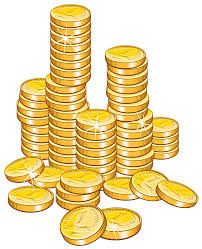Did you find an error or inaccuracy? Feel free to write us. Thank you!Tips to related online calculators
Need help to calculate sum, simplify or multiply fractions? Try our fraction calculator.

## Related math problems and questions:

• There 14There are 250 people in a museum. 2/5 of the 250 people are girls 3/10 of the 250 people are boys The rest of the 250 people are adults Work out the number of adults in the museum.
• Coins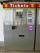In the ticket machine were together one hundred coins. They were only 20 and 50 cent coins. The sum total was 29 euros and 60 cents. How many were in ticket machine coins, and which type?
• RemaindersIt is given a set of numbers { 170; 244; 299; 333; 351; 391; 423; 644 }. Divide this numbers by number 66 and determine set of remainders. As result write sum of this remainders.
• ConcertOn a Concert were sold 150 tickets for CZK 360, 235 tickets for 240 CZK and 412 for 180 CZK. How much was the total revenues for tickets?
• Conference 29 people attended a conference on behalf of their company. The conference fee was £520 per person and the company paid a total of £856 in travel costs for the 9 people. How much did the conference cost the company altogether?
• RatiosReduce the numbers: 50 in a 1:2 ratio 111 at a ratio of 2:3 70 at 10:50 560 at a ratio of 3:8
• DescribedCalculate perimeter of the circle described by a triangle with sides 478, 255, 352.
• Cash back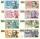After a trip 24 students have left more than 650 but less than 690 CZK (Kc). How much each student receives return when the amount is divisible by the number of pupils?
• RectanglesHow many different rectangles with sides integers (in mm) have a circumference exactly 1000 cm? (a rectangle with sides of 50cm and 450cm is considered to be the same as a rectangle with sides of 450cm and 50cm)
• Four prismsQuestion No. 1: The prism has the dimensions a = 2.5 cm, b = 100 mm, c = 12 cm. What is its volume? a) 3000 cm2 b) 300 cm2 c) 3000 cm3 d) 300 cm3 Question No.2: The prism base is a rhombus with a side length of 30 cm and a height of 27 cm. The height of t
• I think numberI think number.When I add 841 to it and subtract 157, I get a number that is 22 greater than 996. What number I thinking?
• Czech coins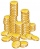John has 540 CZK, but he has 10 CZK coins and 20 CZK coins only. We know that he has 10CZK coins 7-times more than 20CZK coins. How many 10CZK coins and how many 20CZK coins did he save?
• The kitchenThe kitchen has the shape of a square with a side of 5 m. We place linoleum on the floor, the price of which is 450 CZK per 1 m2. The glue costs 750 CZK. How much do we pay in total?
• A bookA book contains 524 pages. If it is known that a person will select any one page between the pages numbered 125 and 384, find the probability of choosing the page numbered 252 or 253.
• Saving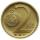Paul saves 155Kč in 46 2 Kc and 5Kc coins. How much saved 2Kc and 5Kc coins?
• Kilo candyOne kilogram of chocolate candy cost 10 euros. Kate buys 250 grams and had the 8 pack of candy. How much does cost one bonbon?
• Czech crownsOldrich has one crown. Peter has five crowns coin, a two crown coin and a one-crown coin. Radek has twenty-crown banknote, ten banknote and five-crown coin. The boys got one fifty-one crown and one crown coin. How can they share the money fairly when they